Author: Oscar Cronquist Article last updated on June 21, 2020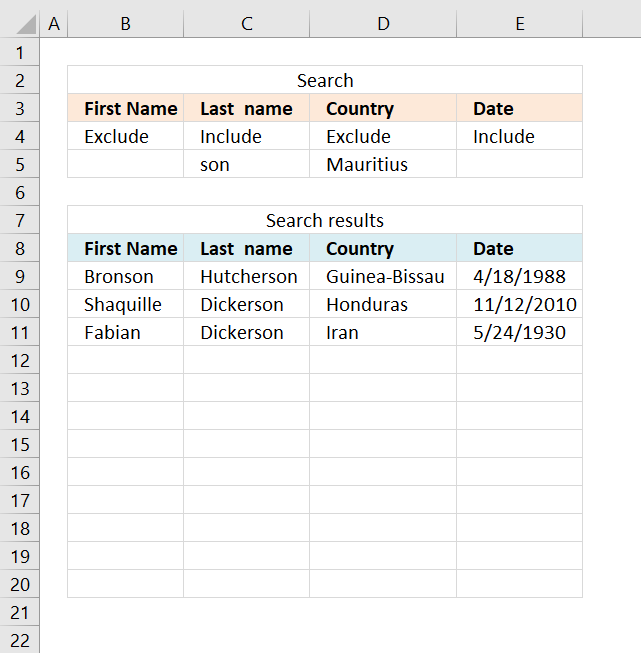This article demonstrates three different ways to filter a data set if a value contains a specific string and if a cell is not equal (exclude) to a specific value. The image above shows an array formula in cell range B9:E20 that extracts values based on the conditions on row 5 and the drop-down lists on row 4.

Hi Oscar,

This is great and compliments your Multiple Criteria and Multiple Matches series of posts very well. However, I have been trying to modify the array formulas in the 3rd and 4th posts of that series to cope with wildcard searches or part cell matches and thought this post may help but not so far, unfortunately.

I have tried numerous approaches but can't seem to get a multiple criteria series of "searches" (or counts) to work with anything but exact data.

I'm trying to search for part of a serial number e.g.(A110 within a string A110E12694369020 as one of my search inputs. In this case, it is always the first 4 digits but I'd like to be able to input just A1 or *A1* into a search cell and not rely on reviewing the first 4 characters as this is not always the result for these serial numbers.

I'm also looking for items with corresponding dates equal or before a certain due date search string - your 4th post to Lookup-with-multiple-criteria-and-display-multiple-search-results-using-excel-formula-part-4-john helped me resolve these criteria though.

Finally, I'm trying another 'wildcard' search and to find FSS (or *FSS*) OR the exact inverse of this - so NOT containing FSS (or *FSS*) - and both of these search criteria are proving elusive to me.

All the data I have is in a large table but I'm not sure if I can alter it to be an Excel Table (which might help), as it is in a sheet that is deleted and replaced by an irregular macro from another workbook and this may corrupt a Table setup(?). It can not be a Pivot as the data is in a shared workbook and Pivot Tables do not update when in shared documents - hence the Array solution I am trying to develop. I have successfully named the columns of ranges I need though without losing them on an update of data.

To summarise - I'd like to search by some form of a wildcard; *app* to return a positive result for "apple" and to search for the opposite; have criteria that would exclude "apple" using something like *app*

Although I have around 80 columns I'm just looking to return the serial number in say Column A based on these multiple criteria; Col B <= a date, Col C does NOT contain a wildcard string *whatever*, Col D DOES contain a different wildcard string, Col E EXACTLY equals a third search string etc. I am happy to build up the criteria for more columns beyond this if I can resolve the NOT and the wildcard elements.

I'd really appreciate some further pointers, thanks Oscar!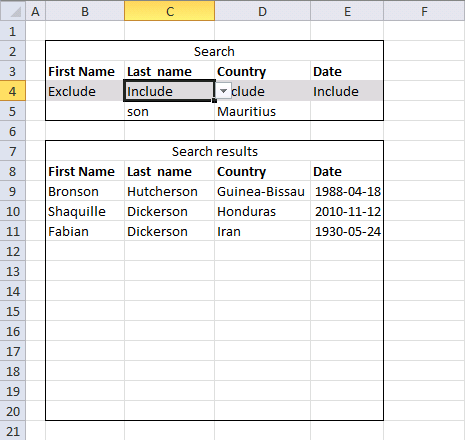Array formula in cell B9:

=IFERROR(IF(COUNTA(\$B\$5:\$E\$5)<>0, INDEX(Sheet2!\$A\$2:\$D\$21, SMALL(IF(COUNTA(\$B\$5:\$E\$5)=((IF(\$B\$4="Include", ISNUMBER(SEARCH(\$B\$5, Sheet2!\$A\$2:\$A\$21)), NOT(ISNUMBER(SEARCH(\$B\$5, Sheet2!\$A\$2:\$A\$21)))))*(\$B\$5<>"")+(IF(\$C\$4="Include", ISNUMBER(SEARCH(\$C\$5, Sheet2!\$B\$2:\$B\$21)), NOT(ISNUMBER(SEARCH(\$C\$5, Sheet2!\$B\$2:\$B\$21)))))*(\$C\$5<>"")+(IF(\$D\$4="Include", ISNUMBER(SEARCH(\$D\$5, Sheet2!\$C\$2:\$C\$21)), NOT(ISNUMBER(SEARCH(\$D\$5, Sheet2!\$C\$2:\$C\$21)))))*(\$D\$5<>"")+(IF(\$E\$4="Include", ISNUMBER(SEARCH(\$E\$5, Sheet2!\$D\$2:\$D\$21)), NOT(ISNUMBER(SEARCH(\$E\$5, Sheet2!\$D\$2:\$D\$21)))))*(\$E\$5<>"")), MATCH(ROW(Sheet2!\$D\$2:\$D\$21), ROW(Sheet2!\$D\$2:\$D\$21)), ""), ROW(A1)), COLUMN(A1)), ""), "")

You don't need to enter this formula as an array formula if you are an Excel 365 subscriber.

#### How to enter an array formula

1. Copy above array formula
2. Select cell B9
3. Paste array formula
4. Press and hold Ctrl + Shift
5. Press Enter

#### How to copy array formula

1. Select cell B9
2. Copy cell B9 (Ctrl + c)
3. Paste to cell range C9:E9
4. Copy cell range B9:E9
5. Paste to cell range B10:E20

### Explaining the formula in cell B9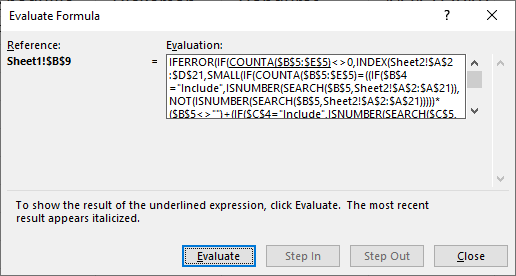I recommend the "Evaluate Formula" tool if you are curious about a formula you don't understand. It is also great for troubleshooting formulas that return errors.

1. Go to tab "Formulas" on the ribbon.
2. Press with left mouse button on "Evaluate Formula" button on the ribbon. A dialog box appears, see image above.
3. Press with left mouse button on "Evaluate" button to see the next calculation step.

The underlined expression shows what part of the formula that will be evaluated next. The italicized is the most recent result.

Keep press with left mouse button oning the "Evaluate button to go through all calculation steps, press with left mouse button on the "Close" button to dismiss the dialog box.

#### Step 1 - Search for a text string

The SEARCH function returns a number representing the position of character at which a specific text string is found reading left to right.

SEARCH(find_text,within_text, [start_num])

SEARCH(\$B\$5, Sheet2!\$A\$2:\$A\$21)

becomes

SEARCH("", {"Jakob"; "Afia"; "Keisha"; "Oliver"; "Roma"; "Frewan"; "Bronson"; "Giovanna"; "Katoka"; "Cyra"; "Shaquille"; "Jakob"; "Ashanti"; "Hamza"; "Gretchen"; "Siena"; "Maggie"; "Fabian"; "Jaydon"; "Derrell"})

and returns

{1; 1; 1; 1; 1; 1; 1; 1; 1; 1; 1; 1; 1; 1; 1; 1; 1; 1; 1; 1}

#### Step 2 - Check if it is a number

The ISNUMBER function checks if a value is a number, returns TRUE or FALSE.

ISNUMBER(value)

ISNUMBER(SEARCH(\$B\$5, Sheet2!\$A\$2:\$A\$21))

becomes

ISNUMBER({1; 1; 1; 1; 1; 1; 1; 1; 1; 1; 1; 1; 1; 1; 1; 1; 1; 1; 1; 1})

and returns

{TRUE; TRUE; TRUE; TRUE; TRUE; TRUE; TRUE; TRUE; TRUE; TRUE; TRUE; TRUE; TRUE; TRUE; TRUE; TRUE; TRUE; TRUE; TRUE; TRUE}

#### Step 3 - Check if cell B4 is "Include" or Exclude"

The IF function returns one value if the logical test is TRUE and another value if the logical test is FALSE.

IF(logical_test, [value_if_true], [value_if_false])

The following part checks if cell B4 equals "Include".

IF(\$B\$4="Include", ISNUMBER(SEARCH(\$B\$5, Sheet2!\$A\$2:\$A\$21)), NOT(ISNUMBER(SEARCH(\$B\$5, Sheet2!\$A\$2:\$A\$21))))

becomes

IF(FALSE, ISNUMBER(SEARCH(\$B\$5, Sheet2!\$A\$2:\$A\$21)), NOT(ISNUMBER(SEARCH(\$B\$5, Sheet2!\$A\$2:\$A\$21))))

becomes

IF(FALSE, {TRUE; TRUE; TRUE; TRUE; TRUE; TRUE; TRUE; TRUE; TRUE; TRUE; TRUE; TRUE; TRUE; TRUE; TRUE; TRUE; TRUE; TRUE; TRUE; TRUE}, {FALSE; FALSE; FALSE; FALSE; FALSE; FALSE; FALSE; FALSE; FALSE; FALSE; FALSE; FALSE; FALSE; FALSE; FALSE; FALSE; FALSE; FALSE; FALSE; FALSE})

and returns

{FALSE; FALSE; FALSE; FALSE; FALSE; FALSE; FALSE; FALSE; FALSE; FALSE; FALSE; FALSE; FALSE; FALSE; FALSE; FALSE; FALSE; FALSE; FALSE; FALSE}

#### Step 4 - Add all arrays

There are four similar parts in the formula described in step 1 to 3, what is different are the cell references. Each part is customized for a column, the list has four columns and the formulas has as many parts as there are columns.

The plus sign applies OR logic to the arrays, for example, 0 + 1 + 0 returns 1. If you multiply the arrays you apply AND logic. 0*1*0 equals 0.

((IF(\$B\$4="Include", ISNUMBER(SEARCH(\$B\$5, Sheet2!\$A\$2:\$A\$21)), NOT(ISNUMBER(SEARCH(\$B\$5, Sheet2!\$A\$2:\$A\$21)))))*(\$B\$5<>"")+(IF(\$C\$4="Include", ISNUMBER(SEARCH(\$C\$5, Sheet2!\$B\$2:\$B\$21)), NOT(ISNUMBER(SEARCH(\$C\$5, Sheet2!\$B\$2:\$B\$21)))))*(\$C\$5<>"")+(IF(\$D\$4="Include", ISNUMBER(SEARCH(\$D\$5, Sheet2!\$C\$2:\$C\$21)), NOT(ISNUMBER(SEARCH(\$D\$5, Sheet2!\$C\$2:\$C\$21)))))*(\$D\$5<>"")+(IF(\$E\$4="Include", ISNUMBER(SEARCH(\$E\$5, Sheet2!\$D\$2:\$D\$21)), NOT(ISNUMBER(SEARCH(\$E\$5, Sheet2!\$D\$2:\$D\$21)))))*(\$E\$5<>""))

becomes

({0;0;0;0;0;0;0;0;0;0;0;0;0;0;0;0;0;0;0;0}+{0;1;0;0;0;0;1;0;0;0;1;0;0;0;0;0;0;1;0;0}+{1;0;1;1;1;1;1;1;1;1;1;1;1;1;1;1;1;1;1;1}+{0;0;0;0;0;0;0;0;0;0;0;0;0;0;0;0;0;0;0;0})

and returns

{1; 1; 1; 1; 1; 1; 2; 1; 1; 1; 2; 1; 1; 1; 1; 1; 1; 2; 1; 1}.

#### Step 5 - Check if the number of criteria matches the array, if it does return the corresponding row number

The COUNTA function counts the non-empty or blank cells in a cell reference.

COUNTA(value1, [value2], ...)

IF(COUNTA(\$B\$5:\$E\$5)=((IF(\$B\$4="Include", ISNUMBER(SEARCH(\$B\$5, Sheet2!\$A\$2:\$A\$21)), NOT(ISNUMBER(SEARCH(\$B\$5, Sheet2!\$A\$2:\$A\$21)))))*(\$B\$5<>"")+(IF(\$C\$4="Include", ISNUMBER(SEARCH(\$C\$5, Sheet2!\$B\$2:\$B\$21)), NOT(ISNUMBER(SEARCH(\$C\$5, Sheet2!\$B\$2:\$B\$21)))))*(\$C\$5<>"")+(IF(\$D\$4="Include", ISNUMBER(SEARCH(\$D\$5, Sheet2!\$C\$2:\$C\$21)), NOT(ISNUMBER(SEARCH(\$D\$5, Sheet2!\$C\$2:\$C\$21)))))*(\$D\$5<>"")+(IF(\$E\$4="Include", ISNUMBER(SEARCH(\$E\$5, Sheet2!\$D\$2:\$D\$21)), NOT(ISNUMBER(SEARCH(\$E\$5, Sheet2!\$D\$2:\$D\$21)))))*(\$E\$5<>"")), MATCH(ROW(Sheet2!\$D\$2:\$D\$21), ROW(Sheet2!\$D\$2:\$D\$21)), "")

becomes

IF(COUNTA(\$B\$5:\$E\$5)={1; 1; 1; 1; 1; 1; 2; 1; 1; 1; 2; 1; 1; 1; 1; 1; 1; 2; 1; 1}, MATCH(ROW(Sheet2!\$D\$2:\$D\$21), ROW(Sheet2!\$D\$2:\$D\$21)), "")

becomes

IF(2={1; 1; 1; 1; 1; 1; 2; 1; 1; 1; 2; 1; 1; 1; 1; 1; 1; 2; 1; 1}, MATCH(ROW(Sheet2!\$D\$2:\$D\$21), ROW(Sheet2!\$D\$2:\$D\$21)), "")

becomes

IF(2={1; 1; 1; 1; 1; 1; 2; 1; 1; 1; 2; 1; 1; 1; 1; 1; 1; 2; 1; 1}, {1; 2; 3; 4; 5; 6; 7; 8; 9; 10; 11; 12; 13; 14; 15; 16; 17; 18; 19; 20}, "")

and returns

{""; ""; ""; ""; ""; ""; 7; ""; ""; ""; 11; ""; ""; ""; ""; ""; ""; 18; ""; ""}.

Now we know which records that match the conditions.

#### Step 6 - Find k-th smallest row number

The SMALL function returns the k-th smallest number in the array, it will also ignore text and blanks.

SMALL(IF(COUNTA(\$B\$5:\$E\$5)=((IF(\$B\$4="Include", ISNUMBER(SEARCH(\$B\$5, Sheet2!\$A\$2:\$A\$21)), NOT(ISNUMBER(SEARCH(\$B\$5, Sheet2!\$A\$2:\$A\$21)))))*(\$B\$5<>"")+(IF(\$C\$4="Include", ISNUMBER(SEARCH(\$C\$5, Sheet2!\$B\$2:\$B\$21)), NOT(ISNUMBER(SEARCH(\$C\$5, Sheet2!\$B\$2:\$B\$21)))))*(\$C\$5<>"")+(IF(\$D\$4="Include", ISNUMBER(SEARCH(\$D\$5, Sheet2!\$C\$2:\$C\$21)), NOT(ISNUMBER(SEARCH(\$D\$5, Sheet2!\$C\$2:\$C\$21)))))*(\$D\$5<>"")+(IF(\$E\$4="Include", ISNUMBER(SEARCH(\$E\$5, Sheet2!\$D\$2:\$D\$21)), NOT(ISNUMBER(SEARCH(\$E\$5, Sheet2!\$D\$2:\$D\$21)))))*(\$E\$5<>"")), MATCH(ROW(Sheet2!\$D\$2:\$D\$21), ROW(Sheet2!\$D\$2:\$D\$21)), ""), ROW(A1))

becomes

SMALL({""; ""; ""; ""; ""; ""; 7; ""; ""; ""; 11; ""; ""; ""; ""; ""; ""; 18; ""; ""}, ROW(A1))

The ROW function returns the row number from the cell reference A1. A1 is a relative cell reference meaning it will change automatically when you copy the cell and paste it to adjacent cells.

This will ensure that only one value is returned per cell.

SMALL({""; ""; ""; ""; ""; ""; 7; ""; ""; ""; 11; ""; ""; ""; ""; ""; ""; 18; ""; ""}, ROW(A1))

becomes

SMALL({""; ""; ""; ""; ""; ""; 7; ""; ""; ""; 11; ""; ""; ""; ""; ""; ""; 18; ""; ""}, 1)

and returns 7.

#### Step 7 - Return value from table

The INDEX function returns a value from a cell range based on a row and column number.

INDEX(Sheet2!\$A\$2:\$D\$21, SMALL(IF(COUNTA(\$B\$5:\$E\$5)=((IF(\$B\$4="Include", ISNUMBER(SEARCH(\$B\$5, Sheet2!\$A\$2:\$A\$21)), NOT(ISNUMBER(SEARCH(\$B\$5, Sheet2!\$A\$2:\$A\$21)))))*(\$B\$5<>"")+(IF(\$C\$4="Include", ISNUMBER(SEARCH(\$C\$5, Sheet2!\$B\$2:\$B\$21)), NOT(ISNUMBER(SEARCH(\$C\$5, Sheet2!\$B\$2:\$B\$21)))))*(\$C\$5<>"")+(IF(\$D\$4="Include", ISNUMBER(SEARCH(\$D\$5, Sheet2!\$C\$2:\$C\$21)), NOT(ISNUMBER(SEARCH(\$D\$5, Sheet2!\$C\$2:\$C\$21)))))*(\$D\$5<>"")+(IF(\$E\$4="Include", ISNUMBER(SEARCH(\$E\$5, Sheet2!\$D\$2:\$D\$21)), NOT(ISNUMBER(SEARCH(\$E\$5, Sheet2!\$D\$2:\$D\$21)))))*(\$E\$5<>"")), MATCH(ROW(Sheet2!\$D\$2:\$D\$21), ROW(Sheet2!\$D\$2:\$D\$21)), ""), ROW(A1)), COLUMN(A1))

becomes

INDEX(Sheet2!\$A\$2:\$D\$21, 7, COLUMN(A1))

The COLUMN function returns the column number from the cell reference A1. A1 is a relative cell reference meaning it will change automatically when you copy the cell and paste it to adjacent cells.

becomes

INDEX(Sheet2!\$A\$2:\$D\$21, 7, 1)

and returns "Bronson" in cell B9.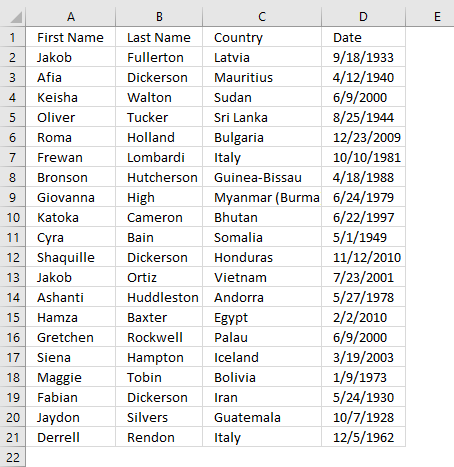The image above shows the data source on worksheet sheet2. Bronson is found on relative row 7 in cell range Sheet2!\$A\$2:\$D\$21.

### Filter tool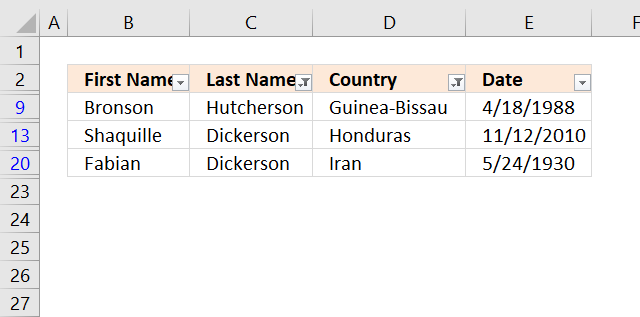The Filter tool built-in to Excel is mostly used to filter a list based on conditions. The difference between the Filter tool and the formulas is that there are a few more extra steps to apply conditions.

The formulas are perhaps harder to enter and customize, however, once they are there they are extremely easy to use.

I will in this tutorial describe how to filter a list based on if a cell contains a specific text string and another column does not equal a specific value. Here are the steps to apply a filter using the Filter tool.

1. Press with left mouse button on any cell in your data with the left mouse button.
2. Go to tab "Data" on the ribbon.
3. Press with left mouse button on the "Filter" button. Black arrows appear next to each column header name.
4. Press with left mouse button on an arrow next to a column you want to filter.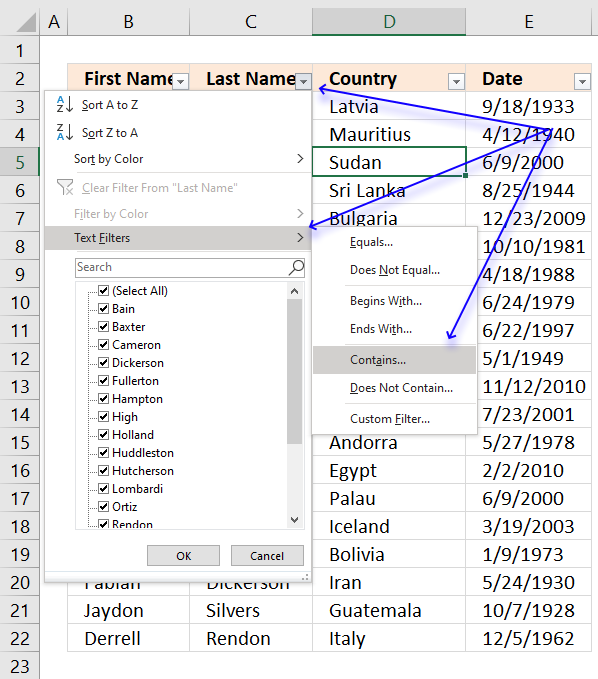5. Press with left mouse button on "Text Filters".
6. Press with left mouse button on "Contains...". A dialog box appears.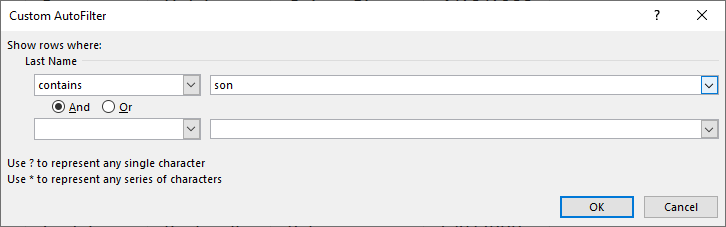7. Type "son", see image above. This will filter the list if values in column name "Last name" contains "son".
8. Press with left mouse button on OK button.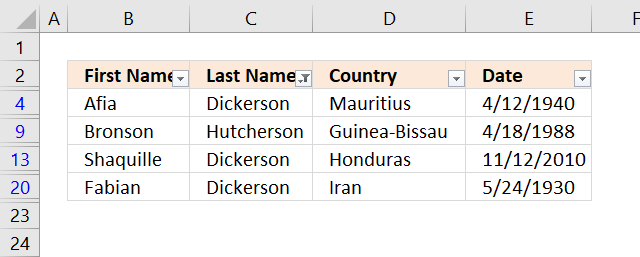The image above shows the list after applying the first condition. Now on to the next condition.

1. Press with left mouse button on the arrow next to the column name "Country".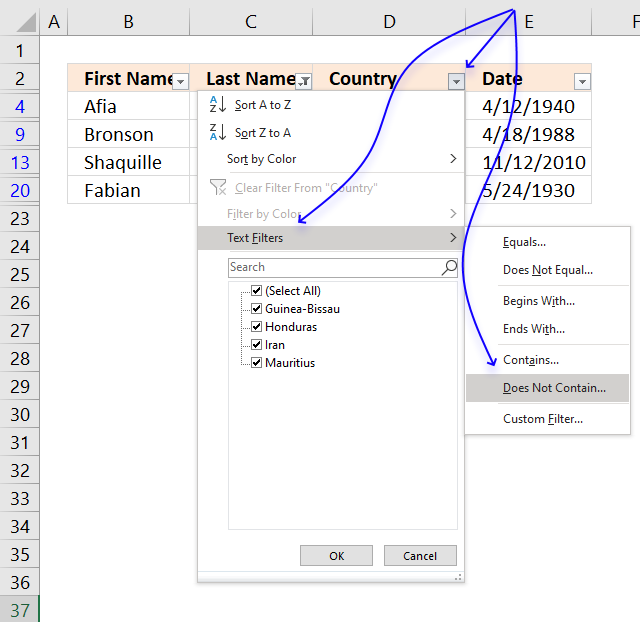2. Press with left mouse button on "Text Filters", see image above.
3. Press with left mouse button on "Does Not Contain...". A dialog box appears.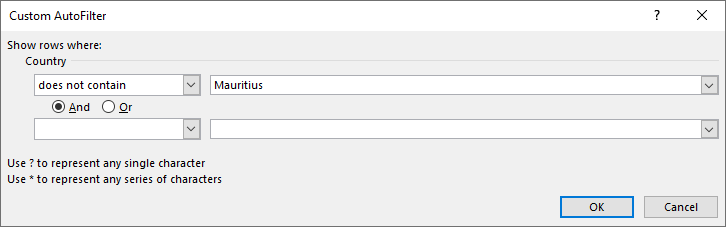4. Type "Mauritius", see image above.
5. Press with left mouse button on OK button.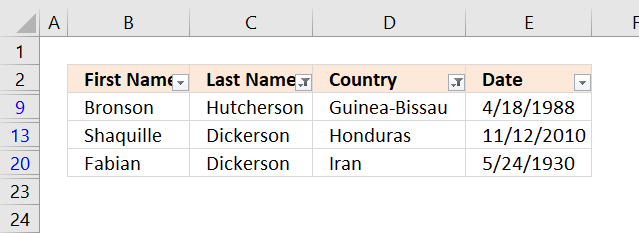### Get the Excel fileLookup-and-include-or-exclude-criteria.xlsx